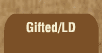Mathematics and Dyslexia

Tips for Learning the Times Tables

Tools for the Times Tables & Workbook

Home > Math > times tables practice > Sixes (small) scrambled

## The Six Times Tables (first set, scrambled)

Remember, if 3 *and* two both go into a number, six does.

1. 6 x 2=
2. 3 x 6=
3. 6 x 1=
4. 6 x 3=
5. 6 x 5=
6. 4 x 6=
7. 5 x 6=
8. 2 x 6=
9. 3 x 6=
10. 6 x 4=
11. 6 x 2=
12. 2 x 6=
13. 6 x 4=
14. 4 x 6=
15. 5 x 6=